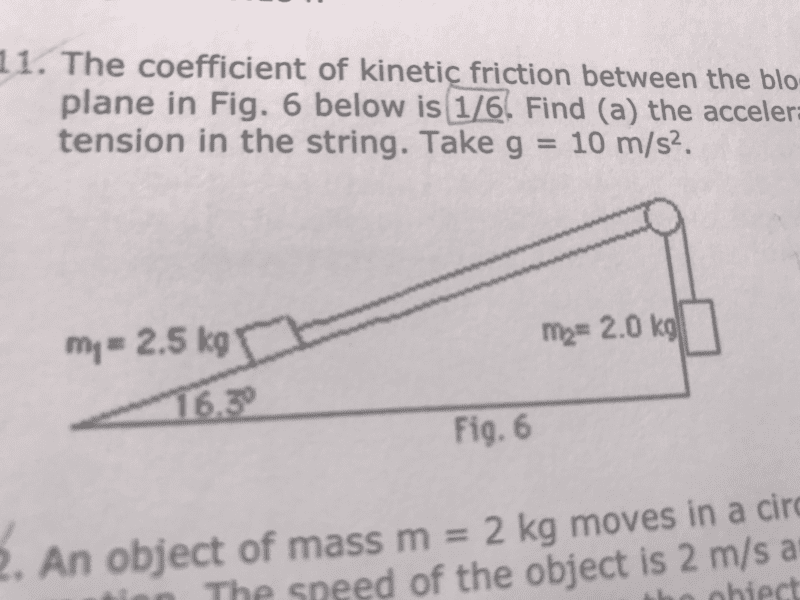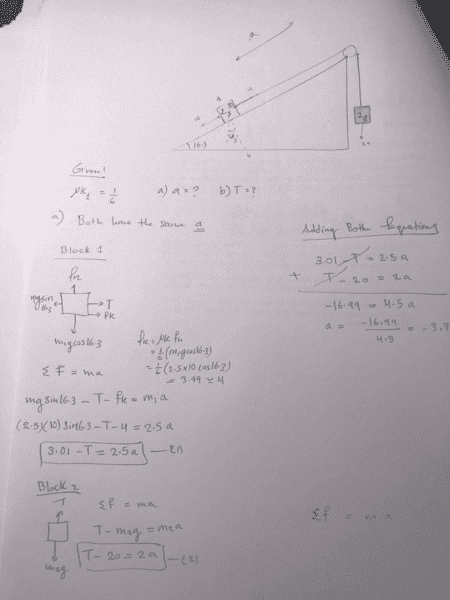# Incline with friction and two blocks

## Homework Statement

The coefficient of kinetic friction between the block of mass m1 = 2.5 kg and the plane in Fig. 6 below is 1/6. Find (a) the acceleration of the block and (b) the tension in the string. Take g = 10 m/s2.Fnet=ma

## The Attempt at a Solution

please i don't know what is the mistake here. the answer should be: a=2m/s^2 .
by the way i am assuming that the orange block is accelerating to the left down across the incline and so the green block up
this is my attempt :i

## Homework Statement

The coefficient of kinetic friction between the block of mass m1 = 2.5 kg and the plane in Fig. 6 below is 1/6. Find (a) the acceleration of the block and (b) the tension in the string. Take g = 10 m/s2.
View attachment 232186

Fnet=ma

## The Attempt at a Solution

please i don't know what is the mistake here. the answer should be: a=2m/s^2 .
by the way i am assuming that the orange block is accelerating to the left down across the incline and so the green block up
this is my attempt :
View attachment 232187
i got a=-3.7 m/s^2

osilmag
Gold Member
I would change the sign of the tension force and the weight (mgsin(theta)) of block 1. Usually "up" the ramp is defined as positive.

•SakuRERE
I would change the sign of the tension force and the weight (mgsin(theta)) of block 1. Usually "up" the ramp is defined as positive.
do you mean that the system will move up right? like hanging block would go down

osilmag
Gold Member
do you mean that the system will move up right? like hanging block would go down

Compare the force of gravity on block 2 to that on block 1 when block 1 is on the ramp. Block 2 should go down.

•SakuRERE
Compare the force of gravity on block 2 to that on block 1 when block 1 is on the ramp. Block 2 should go down.
Thanks i got it just few seconds before you reply•osilmag